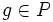# Stability group of subnormal series of finite p-group is p-group

## Statement

Let$P$ be a finite$p$-group, i.e., a group of prime power order where the underlying prime is$p$. Suppose there is a subnormal series:$\{ e \} \triangleleft P_1 \triangleleft P_2 \triangleleft \dots \triangleleft P_n = P$

Consider the subgroup of$\operatorname{Aut}(P)$ given as the group of stability automorphisms of this subnormal series, i.e., as follows:$K := \{ \sigma \in \operatorname{Aut}(P) \mid \sigma(gP_i) = gP_i \ \forall 0 \le i \le n - 1, g \in P_{i+1} \}$

Then$K$ is a$p$-group.

## Related facts

### Converse

A converse of sorts is true for this statement (note that the converse actually establishes a normal series, and not just a subnormal series):

## Facts used

1. Cauchy's theorem: This states that given a finite group, and a prime$q$ dividing the order of the group, there is an element of the finite group of order exactly$q$.

## Proof

### Proof idea

By induction, the proof can be reduced to the case where we have a subnormal series of length two, and an automorphism that fixes both the normal subgroup and the quotient. Next, we iterate this automorphism by its order, and show that the order of the automorphism must equal the order of some element in the normal subgroup, which is hence a prime power.

### Proof details

Given:$P$ is a finite$p$-group, i.e., a group of prime power order where the underlying prime is$p$. Suppose there is a subnormal series:$\{ e \} \triangleleft P_1 \triangleleft P_2 \triangleleft \dots \triangleleft P_n = P$

Consider the subgroup of$\operatorname{Aut}(P)$ given as the group of stability automorphisms of this subnormal series, i.e., as follows:$K := \{ \sigma \in \operatorname{Aut}(P) \mid \sigma(gP_i) = gP_i \ \forall 0 \le i \le n - 1, g \in P_{i+1} \}$

To prove:$K$ is also a$p$-group

Proof: By fact (1), we know that if$K$ were not a$p$-group, we can find elements in it whose order is equal to one of the other prime factors of$K$.

Thus, it suffices to show the following: For any element$\sigma \in K$ such that$\sigma$ has order$m$, relatively prime to$p$,$\sigma$ to be the identity automorphism.

We prove the claim by induction on the order of$P$.$\sigma$ is a stability automorphism for the subnormal series of$P$. Truncating this series at$P_{n-1}$, we get a subnormal series for$P_{n-1}$, and$\sigma$ gives a stability automorphism for that subnormal series as well. Applying the induction assumption to$P_{n-1}$,$\sigma$ gives a stability automorphism of order relatively prime to$p$ on$P_{n-1}$, so$\sigma$ must act as the identity on$P_{n-1}$. Also, by our assumption,$\sigma$ acts as the identity on the quotient$P/P_{n-1}$.

Thus, we need to show that if$\sigma$ acts as identity on$P_{n-1}$ and on$P/P_{n-1}$, and has order$m$ relatively prime to$p$, then$\sigma$ is the identity automorphism.

Pick$g \in P$. Then, consider the element$\sigma(g)$. Clearly, we can write$\sigma(g) = gh$ for some$h \in P_{n-1}$, because$\sigma$ preserves the cosets of$P_{n-1}$. Further, since$\sigma(h) = h$, we have, by induction, that for every positive integer$r$:$\sigma^r(g) = gh^r$

In particular, we have:$g = \sigma^m(g) = gh^m \implies e = h^m$

But$h \in P_{n-1}$ has order a power of$p$, and$m$ is relatively prime to$p$, forcing$h = e$. So$\sigma(g) = g$. This argument works for every$g \in P$, so every element is fixed by the action of$\sigma$ so$\sigma$ is the identity automorphism.